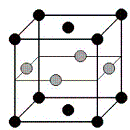## Research Papers in Physics and Astronomy## Ralph Skomski Publications

#### Date of this Version

September 1992

Published in IEEE TRANSACTIONS ON MAGNETICS, VOL. 28. NO. 5, SEPTEMBER 1992.

#### Abstract

The gar phase interstitial modification of rare-earth intermetallics is studied. Net reaction energies for nitrogen in Sm2Fe17 and Nd(Fe11T1) are U0 = - 57 kJ/mole and U0 = - 51 kJ/mole, respectively. The equilibrium nitrogen concentration is calculated as function of temperature and gas pressure using a simple lattice gas model.

For nitrogen in Sm2Fe17, refined diffusion parameters D0 (N) = 1.02 mm2/r and Ea(N) = 133 kJ/mole, determined by thermopiezic analysis of the initial stage of nitrogen absorption, are used to calculate nitrogen profiles and the time dependence of the mean nitrogen content during nitrogenation. Similar values are obtained for nitrogen in Nd (Fe 11Tl), whereas the activation energies for hydrogen in Sm2Fe17 and Nd(Fe11Ti) are 31 kJ/mole and 45 kJ/mole, respectively.

The elastic stress and strain profiles during nitrogenation are calculated. Important results are a large uniaxial strain near the surface of non-uniformly nitrided particles, and core expansion even in the absence of any nitrogen there. Curie temperature and K1 profiles are calculated and suggestions are made regarding the influence of stress on coercivity and disproportionation of the materials.

COinS